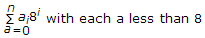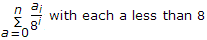# Electronics and Communication Engineering - Microprocessors

### Exercise :: Microprocessors - Section 8

16.

For converting a decimal number into octal number, the decimal number is expressed as a series of the type

 A.B.C.D. None of the above

Explanation:

No answer description available for this question. Let us discuss.

17.

Consider the following statements

1. The sign of a floating point number depends on the sign of mantissa only.
2. If the absolute value of a number is less than 1, the sign of exponent is zero or positive.
3. With sign bit of mantissa as 0, increasing the exponent from a positive to a more positive value gives a larger floating point number.
Which of the above are correct?

 A. All B. 1 and 2 only C. 1 and 3 only D. 2 and 3 only

Explanation:

No answer description available for this question. Let us discuss.

18.

Assertion (A): In microprocessor 8085 instruction LXIB, 90 FF H means register B and C are loaded with upper and lower bytes to get B = 90 H and C = FFH.

Reason (R): In 8085 the stack pointer indicates which memory location is to accessed.

 A. Both A and R are correct and R is correct explanation of A B. Both A and R are correct but R is not correct explanation of A C. A is correct R is wrong D. A is wrong R is correct

Explanation:

No answer description available for this question. Let us discuss.

19.

 A. True B. False

Explanation:

No answer description available for this question. Let us discuss.

20.

The initial contents of `ACC and CY` in 8085 are

1. `CY = 1`
2. `ACC = 10000001`
After instruction `RAL` is executed once the contents of `CY and ACC` respectively will be

 A. 1 and 00000011 B. 0 and 00000011 C. 1 and 00000001 D. 0 and 00000001